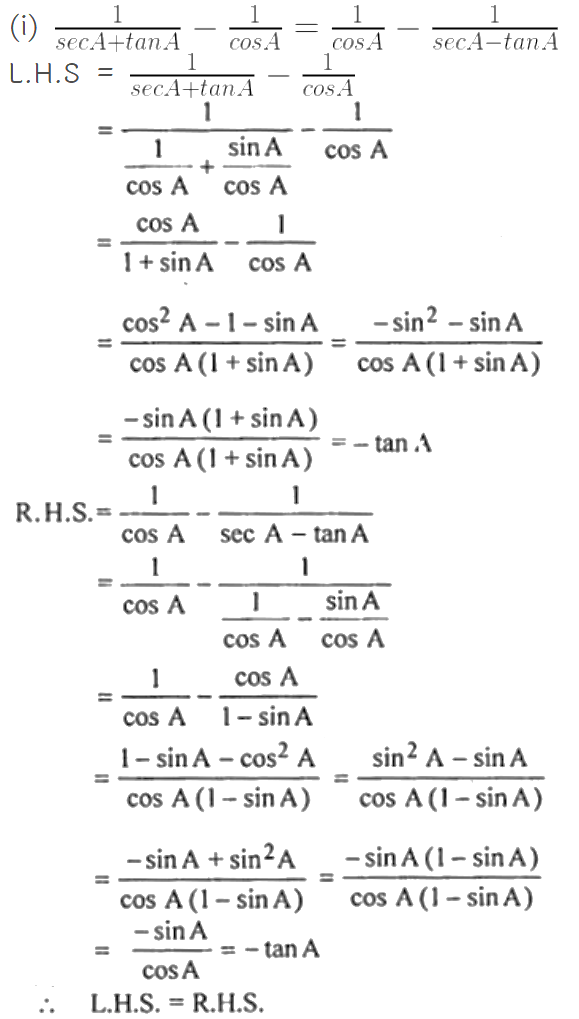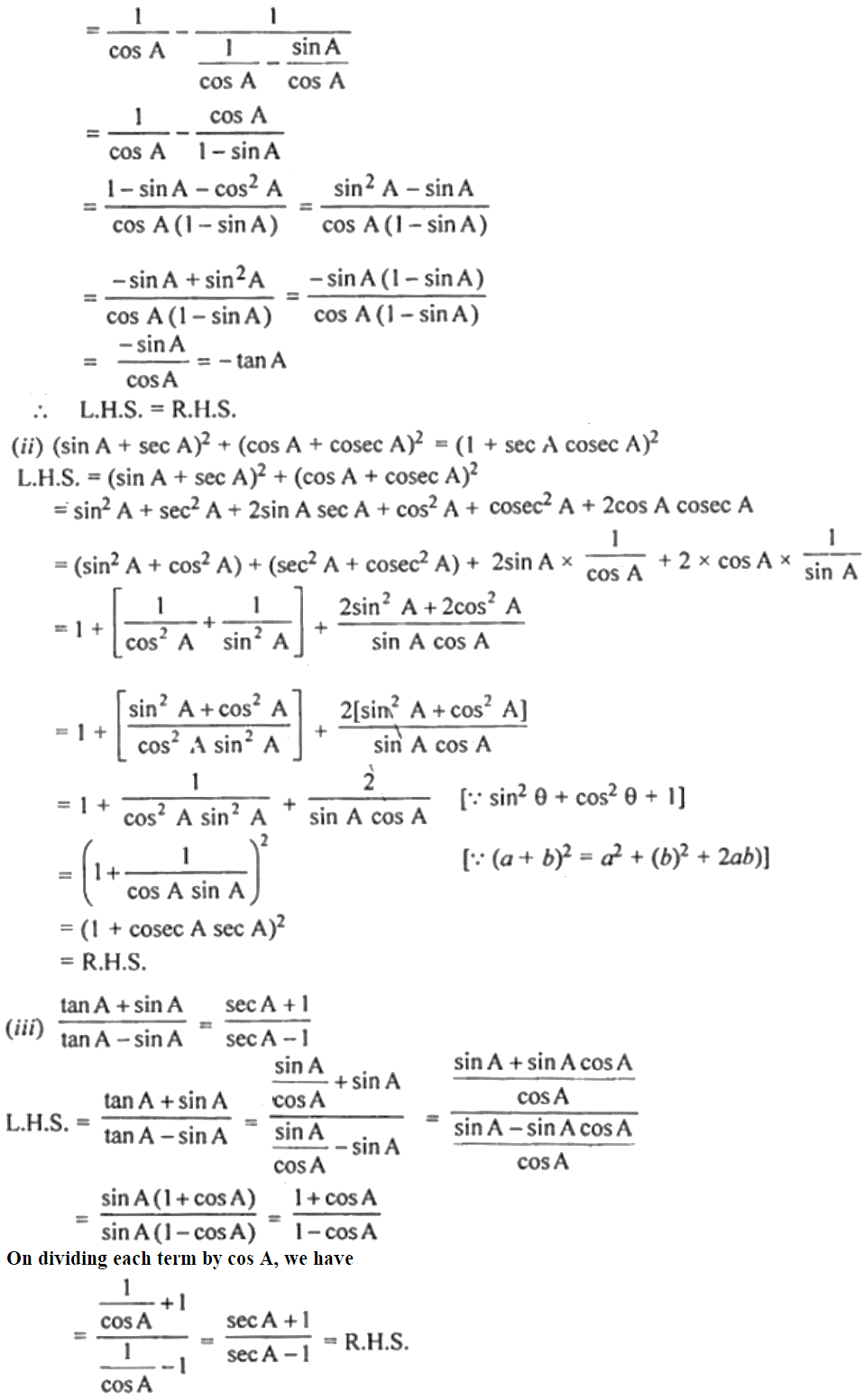Guru

# (i) 1/ (sec A + tan A) – 1/cos A = 1/cos A – 1/(sec A – tan A) (ii) (sin A + sec A)2 + (cos A + cosec A)2 = (1 + sec A cosec A)2 (iii) (tan A + sin A)/ (tan A – sin A) = (sec A + 1)/ (sec A – 1)

• 1

This is an important question from the
Book- ML Aggarwal
Board- ICSE
Publication- Avichal
Chapter- Trigonometric Identities
Chapter number-18

We. Have to prove that

(i) 1/ (sec A + tan A) – 1/cos A = 1/cos A – 1/(sec A – tan A) (ii) (sin A + sec A)2 + (cos A + cosec A)2 = (1 + sec A cosec A)2 (iii) (tan A + sin A)/ (tan A – sin A) = (sec A + 1)/ (sec A – 1)

Trigonometric Identities, class 10th, chapter 18th, question no30 , ICSE board, ML aggarwal..

Share